﻿ An Application of Flower Pollination Algorithm in Model Order Reduction of LTI SystemsPublications are Open
Access in this journal
Article Versions
Export Article
• Normal Style
• MLA Style
• APA Style
• Chicago Style
Research Article
Open Access Peer-reviewed

An Application of Flower Pollination Algorithm in Model Order Reduction of LTI Systems

D. K. Sambariya , Tarun Gupta
American Journal of Electrical and Electronic Engineering. 2018, 6(3), 77-84. DOI: 10.12691/ajeee-6-3-1
Published online: July 26, 2018

Abstract

Representation of physical system using mathematical models leads the process to high-order equations, which are difficult to use for analysis and synthesis. Therefore, it is necessary and useful to find the reduced order equations that describe the same characteristics as the original high-order system. This paper proposes a nature inspired flower pollination algorithm (FPA) for order reduction of original high-order linear time invariant systems. Reduced model will definitely be stable if the original model is stable. Four numerical examples of single-input and single-output systems of different orders are considered to verifying proposed method. The results are compared with different methods available in literature based on step response and performance indices.

1. Introduction

The design of a control system is more complex on the point of analysis, so system is reduced to the simplest model and replaced by the reduced order model. The reduced order model reflects the same characteristics as the original complex model in terms of stability, steady-state error and transient response. There are a number of methods available for model order reduction, and every method reflects some properties of the system. Recently, some have worked on MOR methods are done by different authors such as Kumar 1, describe the classical pade approximation method with different results. Sambariya 2, 3, describes an application of modified Cauer form for MOR of SISO and MIMO linear time invariant systems. Salah 4, has presented an artificial intelligent technique used to find ROM of the high-order system. Narwal 5, has presented the MOR method based on modified clustering algorithm.

Some of the conventional methods are improved Pade approximation 6, 7 by Pal, Continued fraction expansion at general point 8 by Davidson, multipoint Pade approximation 9 by Lucas, multipoint Continued fraction expansion method 10 by Hwang 1986, Cauer continued fraction expansion about two points 11 by Yung, Pade approximation 12 by Pati, Routh stability array method 13 by Sambariya, Error minimization technique 14 by Mukherjee, Factor division method 15 by Lucas, Routh approximation method 16 by Sambariya, Prony-like polynomial-based method 17 by Coluccio, L-J optimization 18 by Luus.

Furthermore a number of mixed methods for MOR are introduced by authors where one is conventional method and second is optimization technique. Clustering Technique and dominant pole method 19 by Agrawal, Clustering technique and frequency response matching 20 by Tiwari, stability equation and big-bang big-crunch optimization 21 by Desai, Eigen spectrum analysis method 22, 23 by Parmar, Mihailov stability criterion and Differential Evolution method 24 by Yadav, Mihailov stability criterion and particle swarm optimization 25 by Panda, Improved clustering algorithm 26 by Komarasamy, big-bang big-crunch 27 by Philip, Particle swarm optimization 28 by Panda, Simulated annealing and simplex method 29 by Wang. A wide range of applications are available of optimization techniques but for model order reduction method, these are applied to objective functions.

This paper, presents flower pollination algorithm (FPA) method 30, 31 for model order reduction. The numerator and denominator coefficients of ROM are evaluated using proposed FPA method. The statement of problem is considered in section 2. In Section 3, overview of flower pollination algorithm is considered. In section 4, steps for proposed methodology are considered. In section 5, SISO examples are considered for model order reduction and compared to the previous models as in literature followed by references. The comparisons are made based on step response characteristics such as settling-time (ts), rise-time (tr), peak, peak-time (tp) and performance indices such as integral square error (ISE), integral absolute error (IAE) and integral time absolute error (ITAE).

2. Statement of Problem Formulation

Consider a higher order transfer function 32 represented in Eq. (1).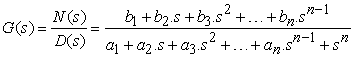(1)

Where,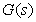represents a high-order transfer function. The high-order transfer function is reduced to low order transfer function. The low order transfer function may be represented in Eq. (2).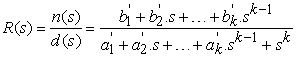(2)

Where,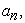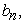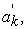and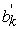are the scalar coefficients of denominator and numerator of high-order transfer functionand reduced-order transfer function_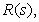respectively.

3. Overview of Flower Pollination Algorithm

3.1. Flower Pollination

Pollination process is finding in nature performed by the flowering plant where pollen of a flowering plant is transfer to the other flowering plant of same species. The pollen transfer process is performed by the small birds, insects etc. The optimization technique based on FPA is first introduced by Xin-She Yang 30. Where author presented the performance of FPA based on number of iterations performed on different functions.

Pollination is the process where pollens of a flower transferred over long distance for reproduction process. The pollinators play important role to transfer pollens of a flower. The pollinators such as birds, insects, bees, flies help to transfer pollens over long distance. Local and Global pollination are the two major types of FPA.

Local pollination or self-pollination or abiotic, there is no requirement of pollinators. Pollination performed by the fertilization of a flower, by air or by diffusion of water. The pollens are transfer to the flower of the same population of the same flowering plant species. Global pollination or cross-pollination performed by using pollinators. Pollinators can travel over long distance to transfer flower pollens. Flower pollens are responsible for reproduction of new flowers or seeds or fruits. Global pollination gives the fittest product than local pollination.

3.2. Flower Pollination Algorithm

The above characteristics point out to some key points in the optimization process. The flower pollination algorithm can be idealized by the following rules 30

1). Pollens transferred by pollinators by the biotic and cross-pollination process. Pollinators contribute in the global pollination where they perform lévy flights.

2). Abiotic and self-pollination are included in local pollination.

3). Reproduction probability is proportional to the similarity of two flowers of same species. This depends on the constancy of a pollinator.

4.) The number of iterations of the global and local pollination can be control by the switch probability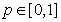. The factors have a significant fraction_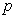in local pollination.

These rules play the important role in mathematical modeling of FPA.

3.3. Mathematical Modeling

In FPA, there are global pollination and local pollination are the two important points in consideration as key points. Rule (1) and (3) are considers in global pollination process. Pollen carrying pollinators (i.e. insects) can travel and move to over long distance, which increases the probability to reproduce fittest, and this represented by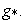This characteristic represented by mathematically as in Eq. (3)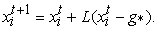(3)

Lévy flight distribution 30 is used to define the step size valid for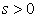and shown by Eq. (4)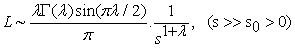(4)

Where,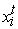= solution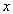from pollen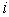at iteration_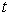,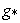= current best solution among all the solutions,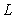= step size which describe the pollination strength,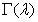= Standard gamma function and we used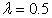.

Rule (2) and (3) are considered in local pollination and this described by Eq. (5)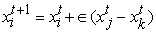(5)

Where,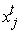and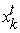are the different flower pollens of same population and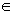defined by uniform distribution in range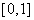._is the neighbor flower pollen of theflower pollen, this neighborhood constancy is considered in this distribution.

There are possibility that flower pollination achieved in both global pollination and local pollination. In this case (Rule (4)), nearby flowers are pollinated by the local flower pollination than that are far away. This condition controlled by using switch probabilityto switch between global pollination and local pollination.

The overall process can describe by the pseudo code as shown in Table 1.

4. Steps for Proposed Methodology

This paper describes an application of FPA method in model order reduction. The numerator and denominator coefficients of ROM are evaluated by minimizing the integral square error (ISE) between the original high-order system and reduced order system. To reduced the given high-order system, the flow chart as in Figure 1 and steps are as followed-

• Figure 1. Flowchart for Flower pollination algorithm

Step a: Evaluate the numerator and denominator coefficients using flower pollination algorithm for reduced second order system.

Step b: Create the following objective function which is used to minimize for SISO system followed by Eq. (6) and fitness of this function is describe by Eq. (7).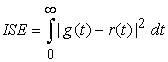(6)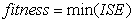(7)

Step c: Check if the fitness function is minimized then gives the numerator and denominator coefficients of ROM else go to step a.

Step d: The ROM of second order will represent by following Eq. (8)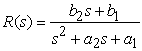(8)

Where,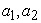and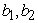are the denominator and numerator unknown coefficients of the reduced second order system respectively. Performance indices such as IAE and ITAE also take in consideration with ISE for comparison. Representation of IAE and ITAE shown in Eq.(9) and Eq.(10) respectively.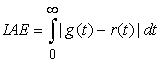(9)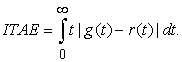(10)

5. Considered Examples and Results

Example-1. Consider a third-order system 1 represented by the transfer function shown in Eq. (11)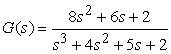(11)

System in Eq. (11) has the characteristics such as poles of system are -2, -1, -1,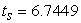,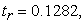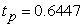and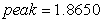. Therefore, the reduced second-order transfer function using proposed FPA method is given by Eq. (12).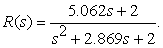(12)

Fitness function describes the performance of search variable using FPA for ROM of Example-1 shown in Figure 2. Step response comparison of original system Eq. (11), ROM by FPA method Eq. (12) and methods in literature for Example-1 shown in Figure 3. Simultaneously, comparison of proposed ROM with different methods tabulated in Table 2 and Table 3. Table 2 shows the comparison based on step response parameters such as settling-time (ts), rise-time (tr), peak and peak-time (tp). On other hand, Table 3 shows the comparison based on performance indices such as ISE, IAE and ITAE between proposed ROM and methods in literature.

• Figure 2. Performance of FPA with minimizing of ISE for ROM of Example-1
• Figure 3. Step response comparison of original system, proposed ROM and other methods in literature for Example-1

Example-2. Consider a forth order system 19 represented by transfer function shown in Eq.(13)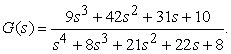(13)

System in Eq. (13) has the characteristics such as poles of system are -4, -2, -1, -1,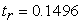,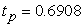and peak = 2.0982. Therefore the reduced second-order transfer function using proposed method is given by Eq. (14).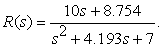(14)

Fitness function describes the performance of search variable using FPA for ROM of Example-2 shown in Figure 4. Step response comparison of original system Eq. (13), ROM by FPA method Eq. (14) and methods in literature for Example-2 shown in Figure 5 and Figure 6. Simultaneously, comparison of proposed ROM with different methods tabulated in Table 4 and Table 5. Table 4 shows the comparison based on step response characteristics such as settling-time (ts), rise-time (tr), peak and peak-time (tp). On other hand, Table 5 shows the comparison based on performance indices such as ISE, IAE and ITAE between proposed ROM and methods in literature.

• Figure 4. Performance of FPA with minimizing of ISE for ROM of Example-2
• Figure 5. Step response comparison of original system, proposed ROM and other methods in literature for Example-2
• Figure 6. Step response comparison of original system, proposed ROM and other methods in literature for Example-2

Example-3. Consider a forth-order system 5 represented by following transfer function shown in Eq. (15)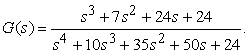(15)

System in Eq. (15) has the characteristics such as poles of system are -4, -3, -2, -1,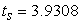,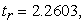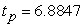and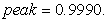Therefore the reduced second-order transfer function using proposed method is given by Eq. (16)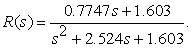(16)

Fitness function describes the performance of search variable using FPA for ROM of Example-3 shown in Figure 7. Step response comparison of original system Eq. (15), ROM by FPA method Eq. (16) and methods in literature for Example-3 shown in Figure 8 - Figure 10. Simultaneously, comparison of proposed ROM with different methods tabulated in Table 6 and Table 7. Table 6 shows the comparison based on step response characteristics such as settling-time (ts), rise-time (tr), peak and peak-time (tp). On other hand, Table 7 shows the comparison based on performance indices such as ISE, IAE and ITAE between proposed ROM and methods in literature.

• Figure 7. Performance of FPA with minimizing of ISE for ROM of Example-3
• Figure 8. Step response comparison of original system, proposed ROM and other methods in literature for Example-3
• Figure 9. Step response comparison of original system, proposed ROM and other methods in literature for Example-3.
• Figure 10. Step response comparison of original system, proposed ROM and other methods in literature for Example-3

Example-4 Consider a fifth-order system 2 represented by following transfer function shown in Eq. (17).(17)

System in Eq. (17) has the characteristics such as poles of system are -4,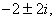-1, -0.5,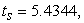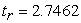,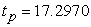and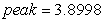. Therefore the reduced second-order transfer function using proposed method is given by Eq. (18).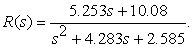(18)

Fitness function describes the performance of search variable using FPA for ROM of Example-4 shown in Figure 11. Step response comparison of original system Eq. (17), ROM by FPA method Eq. (18) and methods in literature for Example-4 shown in Figure 12 – Figure 13. Simultaneously, comparison of proposed ROM with different methods tabulated in Table 8 and Table 9. Table 8 shows the comparison based on step response characteristics such as settling-time (ts), rise-time (tr), peak and peak-time (tp). On other hand, Table 9 shows the comparison based on performance indices such as ISE, IAE and ITAE between proposed ROM and methods in literature.

• Figure 11. Performance of FPA with minimizing of ISE for ROM of Example-4
• Figure 12. Step response comparison of original system, proposed ROM and methods in literature for Example-4
• Figure 13. Step response comparison of original system, proposed ROM and methods in literature for Example-4

6. Conclusion

In this paper, reduced order system from high-order system is obtaining using flower pollination algorithm. Four different orders SISO systems are consider to finding their respective approximations. Proposed FPA method is used to get the reduced order approximations of the high-order system by concept of error minimization. Here ISE is used as objective function to minimize the error between high order and reduced order systems. Comparisons are made based on step responses and performance indices of original system, proposed ROM and different methods available in literature. Performance indices such as ISE, IAE and ITAE values of proposed ROM are compared with different methods in literature. It is found that the proposed ROM shows the better result, performance and almost all the characteristics are preserved.

References

Published with license by Science and Education Publishing, Copyright © 2018 D. K. Sambariya and Tarun GuptaThis work is licensed under a Creative Commons Attribution 4.0 International License. To view a copy of this license, visit http://creativecommons.org/licenses/by/4.0/